# Chemical Bonding and Molecular Structure

## What is Chemical Bond

The attractive force which holds various constituents (atoms, ions, etc.) together in different chemical species is called a chemical bond

## Octet Rule

It states that Atoms of different elements take part in chemical bonding in order to complete their octet or to achieve noble gas configuration.

## Valency

The combining capacity of an atom is known as valency. The elements which give up electrons to form positive ions are said to have positive valency, while the elements which accept electrons to form negative ions are said to have negative valency

## Modes of Chemical Combination: -

a) By sharing of electrons
b) By loss of electrons and gain of electrons
c) Sharing of electrons of single element b/w two atoms.

## Ionic bond / Electrovalent bond

The electrostatic force of attraction between ions formed by loss and gain of electrons
Na   :1s2 2s2 2p6 3s1
Na:1s2 2s2 2p6
Cl : 1s2 2s2 2p6 3s2 3p5
Cl- :1s2 2s2 2p6 3s2 3p6

## Factors affecting Ionic bond

### Electron gain Enthalpy/ Electron Affinity:

Greater the value of Electron gain enthalpy (more negative) easier the formation of anion and more the probability of formation of ionic bond.

### Lattice Energy

It is defined as the amount of energy required to separate one mole of ionic compound into oppositely charged ions separately.
OR
It is the amount of energy required to form ionic compound from one mole of constituent’s ions.
Example
Lattice Energy for Na Cl is -788 KJ/ moles

### Factors affecting Lattice Energy are:

1. Charge of ion -------- More the charge more the force of attraction, Stronger the ionic bond hence higher the lattice energy.
2. Size of ion --------- Smaller the size of ion more the lattice energy.

## Characteristics of Ionic Compounds

1. They generally exists in Crystalline solids having high melting & boiling point.
2. Soluble in polar solvent (mainly water) but do not dissolve in organic solution
3. Poor conductor of electricity (Good conductor in aqueous and fused/ molten state)

## Covalent Bond (Lewis = Longmuir concept)

It is the bond formed by sharing of two or more electrons by different or same atoms.
1. Homoatomic ---- H2, O2, N2, Cl2 etc.
2. Heteroatomic ------ H2 O etc.
If atoms share one pair electron, then it is called single covalent bond. A single covalent bond is represented by a small line (−) between the two atoms.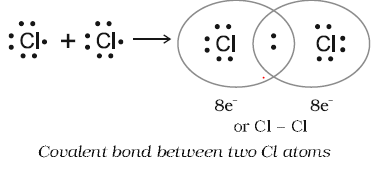If atoms share two pair of electrons, then it is called double covalent bond. A double covalent bond is represented by a small line (=) between the two atoms.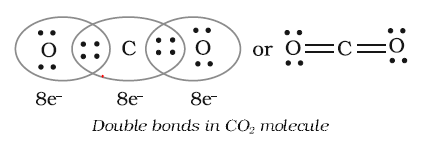If atoms share three pair electrons, then it is called triple covalent bond.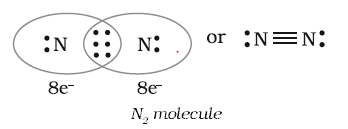## Lewis Dot Structure

It provides the picture of bonds in the molecule and ions in terms of shared number of electron as per the octet rule
Important point to Note
a) Each bond is formed because of sharing of an electron pair between the atoms.
b) Each combining atom contributes at least one electron to the shared pair.
c) The combining atoms attain the outer shell noble gas configurations because of the sharing of electrons.
How to draw it
a) We need to first calculate the valence electron in each of the atom and total sum of them is what will be considered to build the dot structure
b) For anion, each negative charge means addition of electron and for cations, each positive charge means loss of electron
c)  We should arrange electron in such a way that the basic requirement being that each bonded
atom gets an octet of electrons is met.
d) The electrons left after the shared pairs of electrons for bonds are decide are either utilized for multiple bonding or remain as the lone pairs
Note: Lewis dot structures, in general, do not represent the actual shapes of the molecules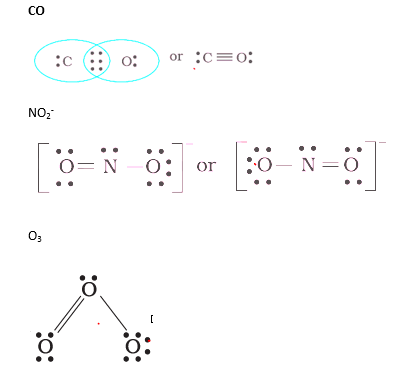## Formal Charge

In polyatomic ions, the net charge on ions is the charge which is residual and not particular for each atom.
The formal charge of an atom in a polyatomic molecule or ion may be defined as the difference between the number of valence electrons of that atom in an isolated or free state and the number of electrons assigned to that atom in the Lewis structure
Formal charge = Total no. of valence Electron - ( No. of lone pair element)  - (1/2)(no. of shared electron)
Example
Formal change on CO2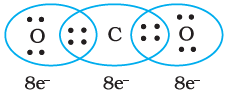Formal charge = Total no. of valence Electron - ( No. of lone pair element)  - (1/2)(no. of shared electron)
Formal change for Ist ‘O’
= 6 – 4 – (1/2)4
= 6 – 6=0
Formal Charge for 2rd O
= 6 – 4 – 2
=0
Formal charge for CO3 -2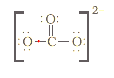For 1st Oxygen atom:-
Formal charge = Total no. of valence Electron - ( No. of lone pair element)  - (1/2)(no. of shared electron)
= 6 – 6 – (1/2) X 2
= 6 – 7 = -1
For 2nd Oxygen atom:-
Fornal charge = 6 – 4 – (1/2) X4
= 6 – 4- 2 = 0
For 3rd oxygen atom:-
Formal charge = 6 – 6 – (1/2) X 2
= 6 – 7 = -1
the lowest energy structure is the one with the smallest formal charges on the atoms. The formal charge is a factor based on a pure covalent view of bonding in which electron pairs are shared equally by neighbouring atoms

## Limitations of Octet Rule

Incomplete Octet
Some of the molecules does not show complete Octet
Example
LiCl, B Cl3, Be H2, etc.
These compounds have incomplete octet yet stable.
Expanded Octet
Example P Cl5
Odd no. of electrons
Example NO2, NO, etc.
Other drawbacks are
(i) Other gases like Xn (Xenon) forms compound like Xe F2, X2F4, X2 O F4, Xe O3, etc.
(ii) It doesn’t give any idea about energy of molecule and stability.
(iii) It doesn’t give any idea about the shape of molecule